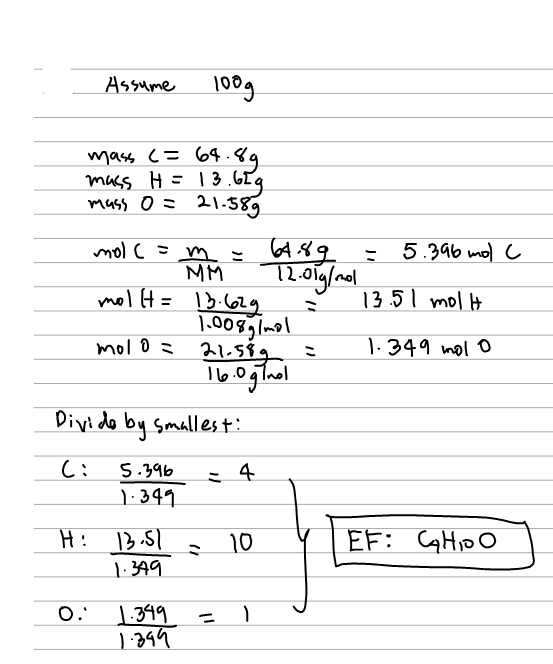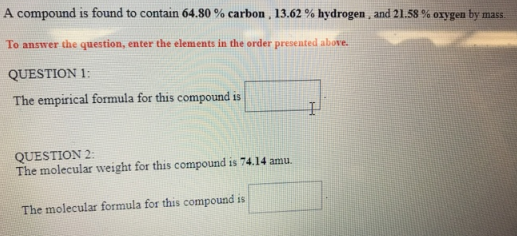# Problem: A compound is found to contain 64.80% carbon, 13.62% hydrogen, and 21.58% oxygen by mass. To answer the question, enter the elements in the order presented above. QUESTION 1:The empirical formula for this compound is __________.QUESTION 2:The molecular weight for this compound is 74.14 amu. The molecular formula for this compound is __________.

###### FREE Expert Solution80% (73 ratings)###### Problem Details

A compound is found to contain 64.80% carbon, 13.62% hydrogen, and 21.58% oxygen by mass.

To answer the question, enter the elements in the order presented above.

QUESTION 1:

The empirical formula for this compound is __________.

QUESTION 2:

The molecular weight for this compound is 74.14 amu.

The molecular formula for this compound is __________.